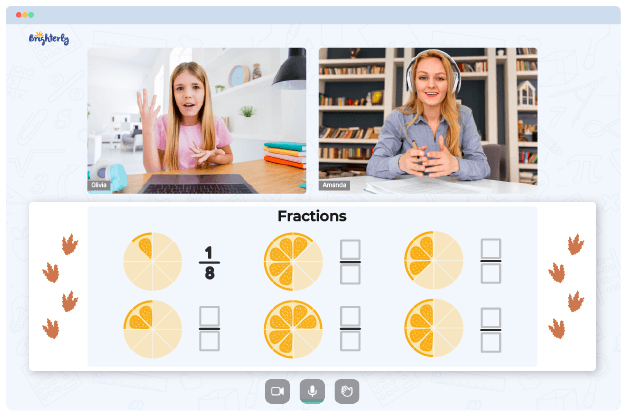# Fractions To Decimals Worksheet

Converting fractions to decimals is a complex topic for kids to grasp. However, like most math topics, fraction conversion gets much more straightforward as kids practice repeatedly. But to truly help expedite the learning process, consider offering your child a change of fractions to decimals worksheet.

## Free Converting Fractions to Decimals Worksheets

Brighterly’s converting fractions to decimals worksheet serves as a basis for students to practice conversion of fractions to decimals. Teachers and parents may use these math manuals at exams, as practical assignments, or as teaching aid to help students improve their arithmetic skills.

When you teach your child to convert fractions to decimals, a worksheet is a good choice as it contains guided exercises and examples that keep children interested in the task. The use of these worksheets is a great way to help your students grasp math concepts quickly. Parents may work with kids to increase practice time or keep math skills sharp during school breaks and even when school is in session.

Math for Kids

Is Your Child Struggling With Math?
1:1 Online Math Tutoring## Benefits of Fraction to Decimal Worksheets

Decimals is a topic that comes in handy in numerous types of calculations. The skill of converting fractions to decimals and vice versa is helpful in everyday life as we deal with decimals when performing financial operations, measuring height, etc. The skill of decimals conversion is a vital one since for example, financial calculations will go awry if you don’t accurately convert fractions to decimals and vice versa.

Due to the importance of the topic, kids may use a fraction to decimal worksheet to get ready for real-world scenarios. Furthermore, they will learn and understand the patterns and logic of mathematics thanks to these worksheets. Over time, kids develop problem-solving abilities and skills to figure out answers logically.

Some platforms also have converting repeating decimals to fractions worksheets that aid in retention of knowledge. As kids practice with worksheets, they will appreciate the uniqueness of the math idea and its application to everyday scenarios.

## Printable Fractions to Decimals Worksheets### Convert Fractions to Decimals (denominators 10, 100 or 1000)

Converting Fractions To Decimals Worksheet### Convert Fractions and Mixed Numbers to Decimals

Fraction To Decimal Worksheet### Convert Mixed Numbers to Decimals

Fractions And Decimals Worksheets### Convert Fractions to Repeating Decimals

Convert Fractions To Decimals Worksheet

To help kids continue learning how to convert fractions into decimals, you can get Brighterly’s printable worksheets. With their fraction to decimal worksheet in PDF format, kids have no excuse not to study as the resources will be accessible at all times. Also, the colorful and engaging format of the worksheets guarantees that kids will learn while having fun.

Converting fractions to decimals can be a tricky process for students. This converting fractions to decimals worksheet is the perfect way for students to practice and master this skill. With multiple levels of difficulty, it allows learners at any level to test their understanding of converting fractions and decimals. Each worksheet consists of several questions in which students have to convert fractions into decimals. Answers are provided so that they can be checked easily. By taking on this converting fractions to decimals worksheet, students will gain the confidence to solve fraction and decimal problems in no time!

Helping your kid with online math lessons for 4th grade. Expert tutors inspiring kids to love math so they can excel at It.

### More Decimals Worksheets

Struggling with Fractions?• Is your child finding it challenging to master fractions?
• An online tutor could be the solution.

Does your child need additional assistance with understanding fractions lessons? Start learning with an online tutor.